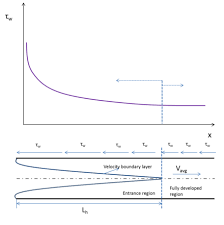## How to Calculate and Solve for Entrance Velocity | Darcy’s Law | Water BudgetThe image above represents entrance velocity.

To compute for entrance velocity, one essential parameters are needed and these parameters are Hydraulic Conductivity (ki).

The formula for calculating entrance velocity:

Ve = ki

Where:

Ve = Entrance Velocity
ki = Hydraulic Conductivity

Let’s solve an example;
Find the entrance velocity when the hydraulic conductivity is 8.\

This implies that;

ki = Hydraulic Conductivity = 8

Ve = ki
Ve = 8

Therefore, the entrance velocity is 8.